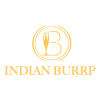# Indian Burrp

Asian Indian Halal

Shop 6/1291-1293 Nepean Highway , Cheltenham, 3192

Delivery from 18:15

HALAL Cuisine : (DF) Dairy Free

-GF = Gluten Free-DF = Dairy Free-V= Vegan, -** = Contains Mix Nuts-* =Contains Cashew Nuts-^v/^df/^gf = Options available (condition apply)

-GF = Gluten Free-DF = Dairy Free-V= Vegan, -** = Contains Mix Nuts-* =Contains Cashew Nuts-^v/^df/^gf = Options available (condition apply)

-GF = Gluten Free-DF = Dairy Free-V= Vegan, -** = Contains Mix Nuts-* =Contains Cashew Nuts-^v/^df/^gf = Options available (condition apply)

-GF = Gluten Free-DF = Dairy Free-V= Vegan, -** = Contains Mix Nuts-* =Contains Cashew Nuts-^v/^df/^gf = Options available (condition apply)

-GF = Gluten Free-DF = Dairy Free-V= Vegan, -** = Contains Mix Nuts-* =Contains Cashew Nuts-^v/^df/^gf = Options available (condition apply)

-GF = Gluten Free-DF = Dairy Free-V= Vegan, -** = Contains Mix Nuts-* =Contains Cashew Nuts-^v/^df/^gf = Options available (condition apply)

-GF = Gluten Free-DF = Dairy Free-V= Vegan, -** = Contains Mix Nuts-* =Contains Cashew Nuts-^v/^df/^gf = Options available (condition apply)

-GF = Gluten Free-DF = Dairy Free-V= Vegan, -** = Contains Mix Nuts-* =Contains Cashew Nuts-^v/^df/^gf = Options available (condition apply)

-GF = Gluten Free-DF = Dairy Free-V= Vegan, -** = Contains Mix Nuts-* =Contains Cashew Nuts-^v/^df/^gf = Options available (condition apply)

-GF = Gluten Free-DF = Dairy Free-V= Vegan, -** = Contains Mix Nuts-* =Contains Cashew Nuts-^v/^df/^gf = Options available (condition apply)

-GF = Gluten Free-DF = Dairy Free-V= Vegan, -** = Contains Mix Nuts-* =Contains Cashew Nuts-^v/^df/^gf = Options available (condition apply)

-GF = Gluten Free-DF = Dairy Free-V= Vegan, -** = Contains Mix Nuts-* =Contains Cashew Nuts-^v/^df/^gf = Options available (condition apply)

-GF = Gluten Free-DF = Dairy Free-V= Vegan, -** = Contains Mix Nuts-* =Contains Cashew Nuts-^v/^df/^gf = Options available (condition apply)